# FSP Semantics

### Syntax of the basic language

A system is described as a hierarchy of interconnected components. Primitive components are computational entities whose behaviour is explicitly defined. The behaviour of composite components is obtained by composing behaviours of their constituent components.

Let:

• L be a set of labels and a, b, c, … range over L
• t be a specific label, corresponding to the internal action
• Act = L È {t} with actions a, b, g, ... ranging over Act
• STOP, ERROR be process constants
• X is a set of variables on processes

We define a set Pr as the smallest set that includes the set of variables X, the process constants STOPA and ERROR, and contains the following expressions, where E, Ei are already in Pr:

1. (a -> E) (prefix)
2. (a1->E1 | a2->E2| …| an->En), where n Î N (choice)
3. (rec X.E) (recursion)
4. E + K, where K Í L (alphabet extension)

The set Prim of primitive process expressions is then defined as:

Prim = {E | (E Î Pr) and (E is closed)}.

An expression E is closed if does not contain free variables, which in this context means all its variables are bound by the recursion operator. As in the case of CSP, we omit brackets in the case of linear sequences of events, on the convention that -> is right associative.

The set Comp of composite process expressions is the smallest set that contains Prim and the following expressions, where E, Ei are already in Comp:

1. E / f , where f is a relabelling function on the set L of labels (relabelling)
2. E @ S, where S Í L (hiding)
3. (E1 || E2) (parallel composition)

As in CSP, the parallel composition operator is commutative and associative which permits the omission of brackets in expressions involving this operator.

### Transitional semantics

We will give meaning to our basic language by using the general notion of a labelled transition system (LTS). Let St be the universal set of states. Formally, an LTS is a quadruple < S, A, D, p > where

1. S Í St is the set of states that are reachable from the initial state p;
2. A = a P È {t}, where a P is the communicating alphabet of P which does not contain the internal action t;
3. D Í S ´ A ´ S, denotes a transition relation that maps from a state and an action onto another state;
4. p is a state in S which indicates the initial state of P.

The set of states reachable from the initial state p is inductively defined as the smallest subset of St satisfying the following conditions:

1. p is reachable
2. if q is reachable and (q, a, q’) Î D, then q’ is reachable.

The operational semantics of Prim is the smallest relation T Í Prim´ L ´ Prim that satisfies the following rules. Rules are given for each of the operators that appear in process expressions. A rule defines the transitions of an expression involving some operator in terms of the transitions of its operands. There will be one or more transition rules associated with each operator. Each rule will have a conclusion and zero or more hypotheses, illustrated as:. In a rule associated with an operator, the conclusion will be a transition of an expression consisting of the operator applied to one or more components, and the hypotheses will be transitions of some of the components. The set of rules associated with each operator can be understood as giving the meaning of that operator.

Prefix: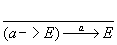Choice: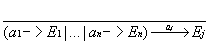, " 0 £ j£ n

Recursion: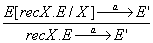Alphabet extension: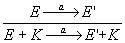The transition relation as defined above can also be used for attributing an alphabet (denoted aE) to each expression E in the following way: if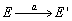then aE = aE’ and a Î aE. In the special case of alphabet extension, a(E + K) = aE È K. Every expression EÎ Prim thus corresponds to an LTS P= S, A, T’, E >, where S Í Prim, A = aE and T’Í T. Finally, we define the two process constants STOP and ERROR as follows:

STOP = < {p}, {}, {}, p} >

ERROR = < {p}, L, {}, p} >.

We have to mention at this point, that the syntax of our basic language Prim does not permit unguarded expressions and therefore all recursive expressions have a unique solution, as demonstrated by Milner in []. Moreover, as only finite choice (and finitely many defining equations, when we will speak about that in the first part) is permitted, our expressions will correspond to LTS that have finite numbers of states. Prim is therefore justified by the fact that we can associate to each primitive process expression an LTS that has a finite number of states. And vice versa, any finite-state LTS can be described by an expression of Prim (this holds for the case where t transitions do not appear in the LTS – such transitions are not needed when describing behaviour of primitive processes. The hiding operator introduced for composite process expressions provides a sufficient extension to cater for these cases).

In a similar way, we will define the semantics of our language Comp of composite expressions by giving meaning to the operators from which they are built.

Relabelling: a(E/f) = {a | a = f(b), b Î a(E)}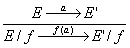Hiding: a(E@S) = a(E)Ç S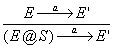, a Î S, a Ï S

Composition: a(P||Q) = a(P)È a(Q), a Ï aQ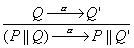, a Ï aP, a ¹ t

We also define the following:

ERROR || P = P || ERROR = ERROR, " P Î Comp,

ERROR @ S = ERROR, " S,

ERROR/f = ERROR, " f.

Expressions in Comp are built up from terms of the language Prim, which are mapped to finite-state LTS. By the transition and alphabet rules described above, terms of Comp are mapped to LTSs in a similar way. It can be easily shown that relabelling and hiding to not affect the number of states of the LTS corresponding to the expression to which they are applied. The composition of expressions that correspond to LTS with sizes s1, s2 respectively, will be mapped to an LTS with size equal to s1x s2, in the worst case (this is because we do not allow recursive expression with respect to the composition operator). We conclude that all expressions in Comp can also be mapped to finite-state LTS.